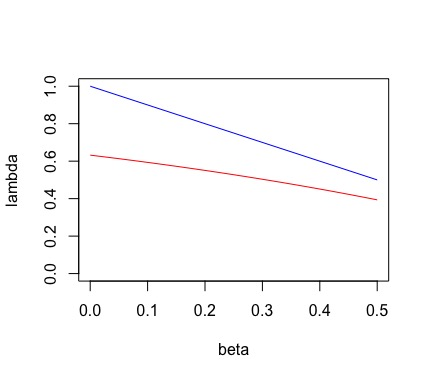Scroll

Categories

Tags

Monthly Archives

Keyword

Back

2019.02.03

# Prism Notes

Prism论文地址

The address of the Prism paper

## 数学符号

$\beta$: the fraction of hashing power the adversary can control without compromising system security, defined in section 1.1.

$\beta$：在系统安全性不受危害的情况下攻击者能控制的哈希算力的部分。定义在1.1节。

$\lambda$: the throughput, number of transactions confirmed per second, defined in section 1.1.

$\lambda$：吞吐量，即每秒钟确认的交易数量。定义在1.1节。

$B$: the number of transactions contained in a block, defined in section 3.1.

$B$：一个区块里包含的交易数量。定义在3.1节。

$C$: the communication capacity of the network, measured in transactions per second, defined in section 1.2.

$C$：网络的通信承载力，以每秒承载的交易数量为度量。定义在1.2节。

$D$: the speed-of-light propagation delay across the network, measured in seconds, defined in section 1.2.

$D$：信息传遍网络所需的光速延迟，以秒度量。定义在1.2节。

$f$: the block mining rate, defined in section 2.1.

$f$：挖矿速率。定义在2.1节。

$\Delta$: the time taken transmitting a block from one honest node to another honest node, measured in seconds; on the other hand, the adversary can transmit and receive blocks with arbitrary delay, up to delay $\Delta$; defined in section 3.1. In the discrete-time round-by-round synchronous model, each round corresponds to $\Delta$ seconds. $\Delta = \frac{B}{C} + D$ (formula (5) in section 3.2).

$\Delta$：将区块从一个诚实节点传送到另一个诚实节点所需的时间，以秒度量；另一方面，攻击者可以以任意时间的延迟来传送和接收区块，延迟上限是$\Delta$。定义在3.1节。 在离散时间回合制同步模型中，每回合对应$\Delta$秒。 $\Delta = \frac{B}{C} + D$（3.2节公式(5)）。

$N$: the set of participating nodes in the network, defined in section 3.1.

$N$：网络中所有节点的集合。定义在3.1节。

$H \subset N$: the honest nodes, who strictly follow the protocol, defined in section 3.1.

$H \subset N$：严格遵循协议的诚实节点的集合。定义在3.1节。

$p_n$: the fraction of total hashing power controlled by node $n$, defined in section 3.1.

$p_n$：节点$n$所控制的哈希算力占所有哈希算力的比例。定义在3.1节。

$H[r]$: the number of blocks mined by the honest nodes in the $r$th round, defined in section 3.1. This variable is Poisson distributed with means $(1 − \beta)f\Delta$.

$H[r]$：第$r$回合中诚实节点挖到的区块数量。定义在3.1节。该变量服从均值为$(1 − \beta)f\Delta$的泊松分布。

$Z[r]$: the number of blocks mined by the adversarial nodes in the $r$th round, defined in section 3.1. This variable is Poisson distributed with means $\beta f\Delta$.

$Z[r]$：第$r$回合中攻击节点挖到的区块数量。定义在3.1节。该变量服从均值为$\beta f\Delta$的泊松分布。

$\overline{\lambda} := \lambda / C$: the throughput as a fraction of the network capacity and is a measure of the efficiency of a protocol, defined in the beginning of section 4.

$\overline{\lambda} := \lambda / C$：网络承载力的一部分，是对协议效率的一种度量。定义在第4节的开头。

$\overline{f}_{\text{BTC}}(\beta)$: the unique solution of $\overline{f}$ to the equation $1 - e^{-(1 - \beta)\overline{f}} = \beta \overline{f}$ (formula (9)), defined in section 4.1.

$\overline{f}_{\text{BTC}}(\beta)$：等式$1 - e^{-(1 - \beta)\overline{f}} = \beta \overline{f}$（公式(9)）中$\overline{f}$的唯一解。定义在4.1节。

$\varepsilon$: In Bitcoin protocol, a current main chain block remains in the future main-chain with probability $1 - \varepsilon$ if on the order of $\log 1/\varepsilon$ successive blocks are mined over it. Defined in section 5.1.

$\varepsilon$：在比特币协议中，当前主链上的一个区块在将来仍然保留在主链中的概率是$1 - \varepsilon$，如果后续大约$\log 1/\varepsilon$个区块是在它的基础上被挖出的话。定义在5.1节。

## 有助于理解的笔记

4.3节推导出的Prism 1.0的吞吐量公式是$\overline{\lambda} = 1 - e^{\beta - 1}$，即下图的红色曲线。 但理想吞吐量公式是$\overline{\lambda}^* = 1 - \beta$，即下图的蓝色曲线。It is written in section 5.1 that

Recall physical limits impose two lower bounds on the latency: (1) the propagation delay $D$, and (2) $1/C \log 1/\varepsilon$

Prism完整版则解决了确认时间的问题。 它进一步将核心区块分为提议区块和投票区块。 1棵提议树和$m$棵投票树。 提议区块只管排序，不管验证；双花的时候也只取它看到的最早的花费。 验证逻辑交给投票区块。 每棵投票树的出块率依然很低，但是$m$很大，$m$棵投票树并行投票的话，确认速度就会变快。

## 问题

Q: Why “one can simply increase the number of transaction blocks that a proposer block points to with- out compromising the security of the blockchain” (mentioned in section 4.1)?

Q: In section 3.1, why the adversary can transmit and receive blocks with arbitrary delay, up to delay $\Delta$?

Q: In section 4.1, why the rate of growth of Bitcoin is a probability (formula (7)) and its maximum is 1?

Q: Why the adversary does not know which type of block it is mining until after the block has been mined?

Q: It is written in section 4.2 that “a node that hears of a block determines its validity by checking the hash”, so what does a node do specifically when it checks the hash to determine the validity of a received block?

Q: What is common-prefix property mentioned in section 4.3?

Q: What is positive chain quality mentioned in section 4.3?

Top